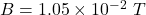## A long solenoid has a length of 0.54 m and contains 1900 turns of wire. There is a current of 2.5 A in the wire. What is the magnitude of th

Question

A long solenoid has a length of 0.54 m and contains 1900 turns of wire. There is a current of 2.5 A in the wire. What is the magnitude of the magnetic field within the solenoid?

in progress 0
5 months 2021-08-28T11:29:38+00:00 1 Answers 2 views 0

Therefore,

Strength magnetic field at within the Solenoid,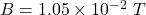Explanation:

Given:

Turn = N = 1900

length of solenoid = l = 0.54 m

Current, I = 2.5 A

To Find:

Strength magnetic field within the Solenoid,

B = ?

Solution:

If N is the number of turns in the length, the total current through the rectangle is NI. Therefore, Ampere’s law applied to this path gives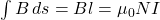Where,

B = Strength of magnetic field

l = Length of solenoid

N = Number of turns

I = Current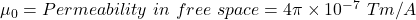Therefore,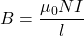Substituting the values we get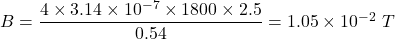Therefore,

Strength magnetic field at within the Solenoid,